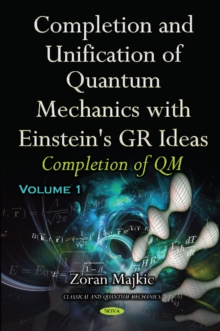Supporting your high street Find out how »
• My Account# Completion & Unification of Quantum Mechanics with Einstein's GR Ideas : Part I -- Completion of QM Hardback

#### Description

This unique manuscript presents a novel approach to QM by modelling an elementary particle via 3D matter/energy density, which propagates in the open time-space continuum as a rest mass energy density wave packet.

This simple idea is based on the fact that any macroscopic object of mass M that occupies a finite 3D volume V can be represented by an energy-density contained in V, so that the integration of this energy-density over V provides the total energy E = Mc^2 .

This new theory is fully integrated with the theory of relativity, and completes the quantum theory of Einstein by overcoming the Copenhagen interpretation.

The newly introduced partial differential equations describe the relativistic phenomena and, generally, the dependence of a particle's geometrical form (its internal matter distribution) on its velocity and acceleration.

A number of well-known physical principles are obtained as derived results of this theory, and are consolidated by a number of detailed examples.

Part I, which is dedicated to the completion of QM, is composed of five Chapters.

In the first two chapters, the nucleus is analysed in terms of the material discussed.

Chapter Three is dedicated to the development of the Lagrangian density for the complex wave packets of the rest mass energy density of an elementary particle, and to the new quantum field theory.

The authors obtained the set of new non-Hamiltonian TSPF quantum operators, parameterised by the vector velocity field of energy density with corresponding Hilbert spaces for accelerated particles, and these were valid in any (infinitesimal) local Minkowski time-space.

The main results are the new differential equations obtained as conservation laws for Noether currents and Euler-Lagrange equations, which express the exact form of the complex terms used in the differential equations in Chapters One and Two, and introduce the most useful concept of the velocity for any infinitesimal amount of the energy density flux of a particle (the hidden variables).

In Chapter Four, a gauge theory and a new explanation of the mass gap conjecture in Yang-Mils theory and of the Higgs mechanism without necessity of the new Higgs field and its bosons, along with a new explanation of double-slit experiments are presented.

Thus, the authors obtained a conservative extension of current probabilistic/statistic QM valid for an ensemble of particles, each individual particle, and which is deterministic and compatible by classical mechanics.

#### Information

£193.99

£155.39

on all orders

###### Pick up orders

from local bookshops

£193.99

£155.39

£193.99

£155.39

£53.99

£45.05

£79.99

£65.65